Linear Algebra (Part - 2)

# Linear Algebra (Part - 2) - Notes | Study Additional Documents & Tests for Civil Engineering (CE) - Civil Engineering (CE)

 1 Crore+ students have signed up on EduRev. Have you?

Question 16: For what value of p the following set of equations will have no solution?    [2015 : 1 Mark, Set-I]
2x + 3y = 5
3x + py = 10
Solution:
Given system of equations has no solution if the lines are parallel i.e., their slopes are equal
2/3 = 3/p
⇒ p = 4.5
Question 17: The rank of the matrix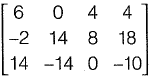is _____.    [2014 : 2 Marks, Set-II]
Solution: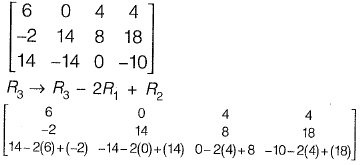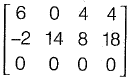Determinant of matrix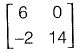is not zero.
∴ Rank is 2
Question 18: The determinant of matrix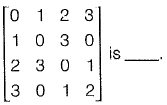[2014 : 1 Mark, Set-II]
Solution: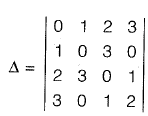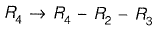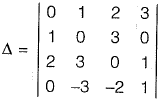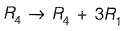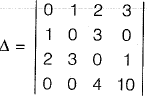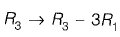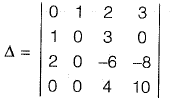Interchanging column 1 and column 2 and taking transpose,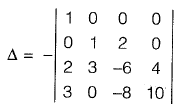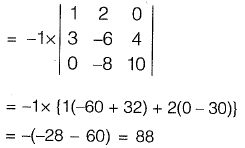Question 19: With reference to the conventional Cartesian (x, y) coordinate system, the vertices of a triangle have the following coordinates; (x1, y1) = (1, 0); (x2, y2) = (2, 2); (x3, y3) = (4, 3). The area of the triangle is equal to    [2014 : 1 Mark, Set-I]
(a) 3/2
(b) 3/4
(c) 4/5
(d) 5/2
Solution: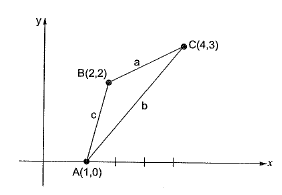Area of triangle is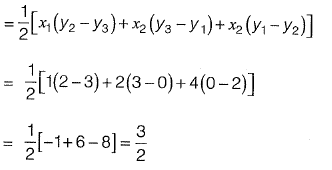Question 20: The sum of Eigen values of matrix, [M] is where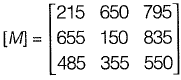[2014 : 1 Mark, Set-I]
(a) 915
(b) 1355
(c) 1640
(d) 2180
Solution: Sum of eigen values = trace of matrix
= 215 + 150 + 550 = 915

Question 21: Given the matrices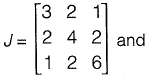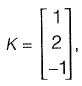the product KT JK is ____.    [2014 : 1 Mark, Set-I]
Solution: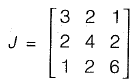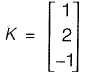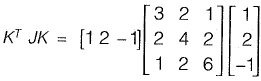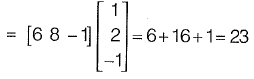Question 22: There are three matrixes P(4 x 2), Q(2 x 4) and R(4 x 1). The minimum of multiplication required to compute the matrix PQR is    [2013 : 1 Mark]
Solution: If we multiply QR first then,
Q2x4 x R(4x1) having multiplication number 8.
There fore P(4 x 2) QR(2 x 1) will have minimum number of multiplication = (8 + 8) = 16.

Question 23: The eigen values of matrix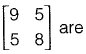[2011 : 2 Marks]
(a) -2.42 and 6.86
(b) 3.48 and 13.53
(c) 4.70 and 6.86
(d) 6.86 and 9.50
Solution: We need eigen values of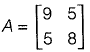The characteristic equation is,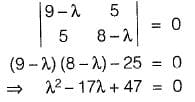So eigen values are,
λ = 3.48, 13.53

Question 24: [A] is square matix which is neither symmetric nor skew-symmetric and [A]T is its transpose. The sum and difference of these matrices are defined as [S] = [A] + [A]T and [D] = [A] - [A]T, respectively. Which of the following statements is TRUE?    [2011 : 1 Mark]
(a) Both [S] and [D] are symmetric
(b) Both [S] and [D] are skew-symmetric
(c) [S] is skew-symmetric and [D] is symmetric
(d) [S] is symmetric and [D] is skew-symmetric
Solution: Since (A + At) = At + (At)t
= At + A
i.e. St = S
∴ S is symmetric
Since (A - At)t = At - (At)t
= At - A = -(A - At)
i.e. Dt = - D
So D is Skew-Symmetric.

Question 25: The inverse of the matrix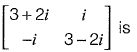[2010 : 2 Marks]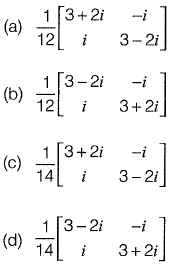Solution: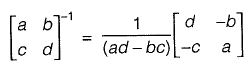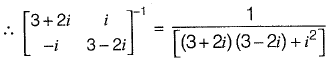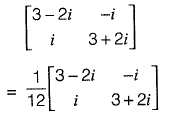The document Linear Algebra (Part - 2) - Notes | Study Additional Documents & Tests for Civil Engineering (CE) - Civil Engineering (CE) is a part of the Civil Engineering (CE) Course Additional Documents & Tests for Civil Engineering (CE).
All you need of Civil Engineering (CE) at this link: Civil Engineering (CE)

## Additional Documents & Tests for Civil Engineering (CE)

64 docs|6 tests
 Use Code STAYHOME200 and get INR 200 additional OFF

## Additional Documents & Tests for Civil Engineering (CE)

64 docs|6 tests

Track your progress, build streaks, highlight & save important lessons and more!

,

,

,

,

,

,

,

,

,

,

,

,

,

,

,

,

,

,

,

,

,

;